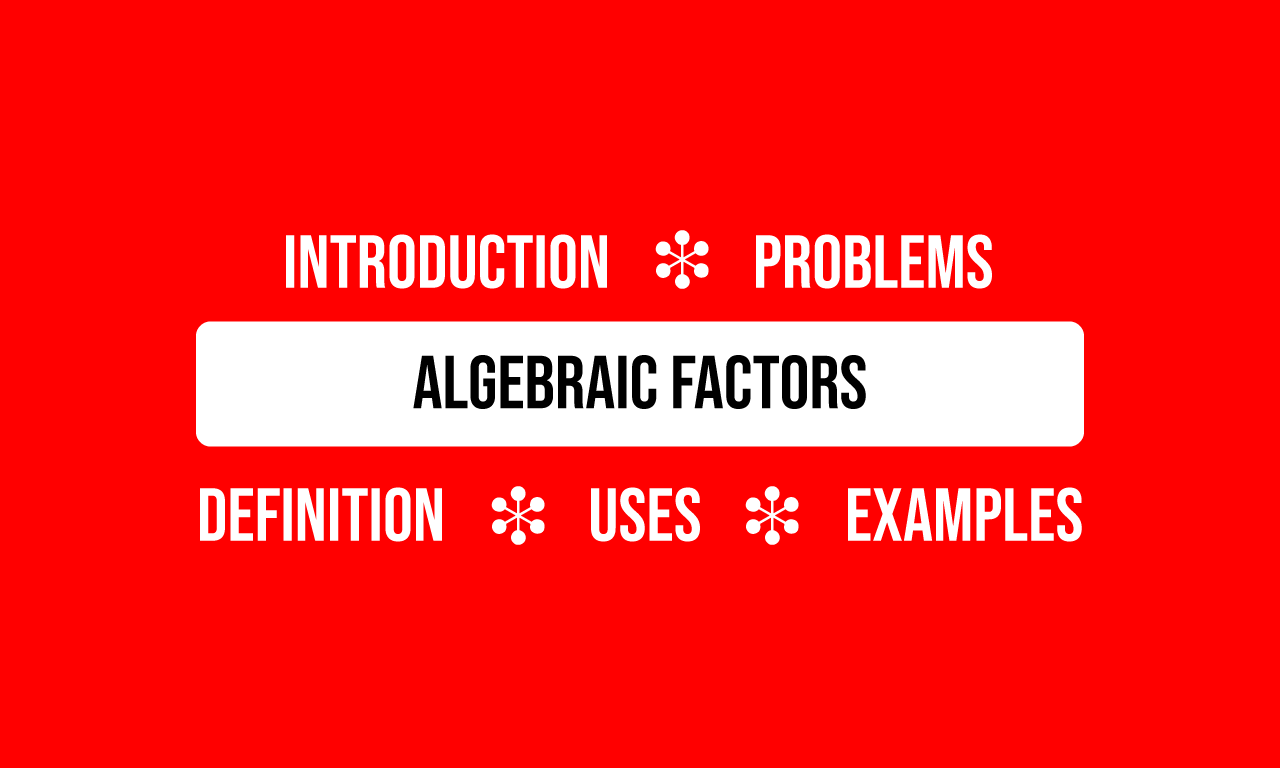# Algebraic factors

A quantity in algebraic form that multiplies at least one quantity in a term of an expression is called an algebraic factor.

## Introduction

A term in an algebraic expression or the whole algebraic expression is simplified as a product of two or more multiplying expressions for some reasons. Each multiplying expression represents a quantity in algebraic form and it is called a factor in algebraic form. It is simply called as an algebraic factor in mathematics.

#### Examples$(1).\,\,\,$ $ax$

$\implies$ $ax$ $\,=\,$ $a \times x$

1. $a$ and $x$ are two literals basically but they express quantities in algebraic form.
2. The two expressions $a$ and $x$ are multiplied each other. So, they are called algebraic factors.

$(2).\,\,\,$ $x^2(x-3)$

$\implies$ $x^2(x-3)$ $\,=\,$ $x^2 \times (x-3)$

1. $x^2$ and $x-3$ are a monomial and a binomial and the two algebraic expressions denote indeterminate quantities.
2. The algebraic expressions $x^2$ and $x-3$ are multiplied each other. Hence, the expressions $x^2$ and $x-3$ are called algebraic factors.

$(3).\,\,\,$ $(x+a)(ax+b)(x^4+7x-c)$

$\implies$ $(x+a) \times (ax+b) \times (x^4+7x-c)$

1. $x+a,$ $ax+b$ and $x^4+7x-c$ are three polynomials, which represent three unknown quantities.
2. The given expression is a product of three expressions $x+a,$ $ax+b$ and $x^4+7x-c$. Therefore, the expressions $x+a,$ $ax+b$ and $x^4+7x-c$ are called algebraic factors.

The above three examples have cleared that an algebraic factor can be a literal, monomial, binomial, trinomial and so on.

#### Uses

There are three advantages with the algebraic factors in mathematics.

1. To express algebraic expressions in simple form.
2. For simplifying the algebraic expressions.
3. To calculate the unknown values of the variables of algebraic expressions.

#### Factorization

An algebraic expression is mathematically defined in either sum or difference or combination of both. However, it should be simplified in factor form in some cases by factorisation. So, it is essential to learn how to factorise (or factorize) the algebraic expressions in mathematics.

In mathematics, there are six methods for factorizing (or factorising) the algebraic expressions. Now, learn each method of factoring the algebraic expressions with understandable steps of solution.

Latest Math Topics
Jun 26, 2023
Jun 23, 2023

Latest Math Problems
Jul 01, 2023
Jun 25, 2023
###### Math Questions

The math problems with solutions to learn how to solve a problem.

Learn solutions

Practice now

###### Math Videos

The math videos tutorials with visual graphics to learn every concept.

Watch now

###### Subscribe us

Get the latest math updates from the Math Doubts by subscribing us.